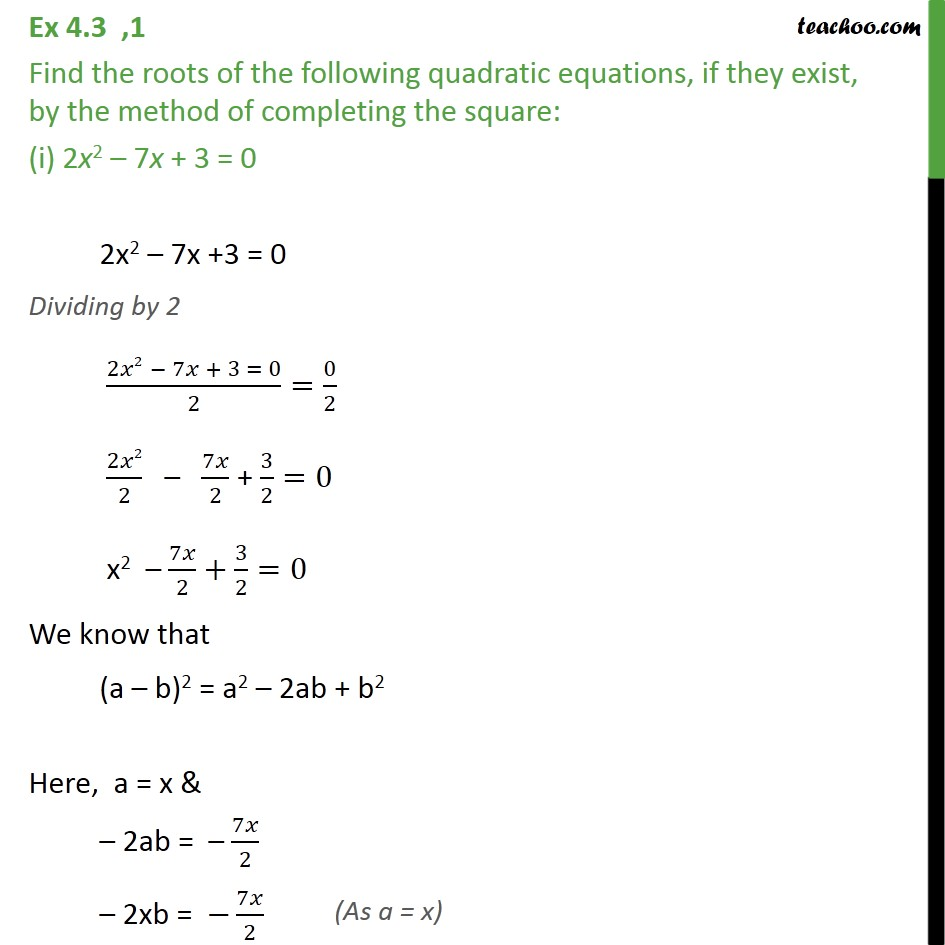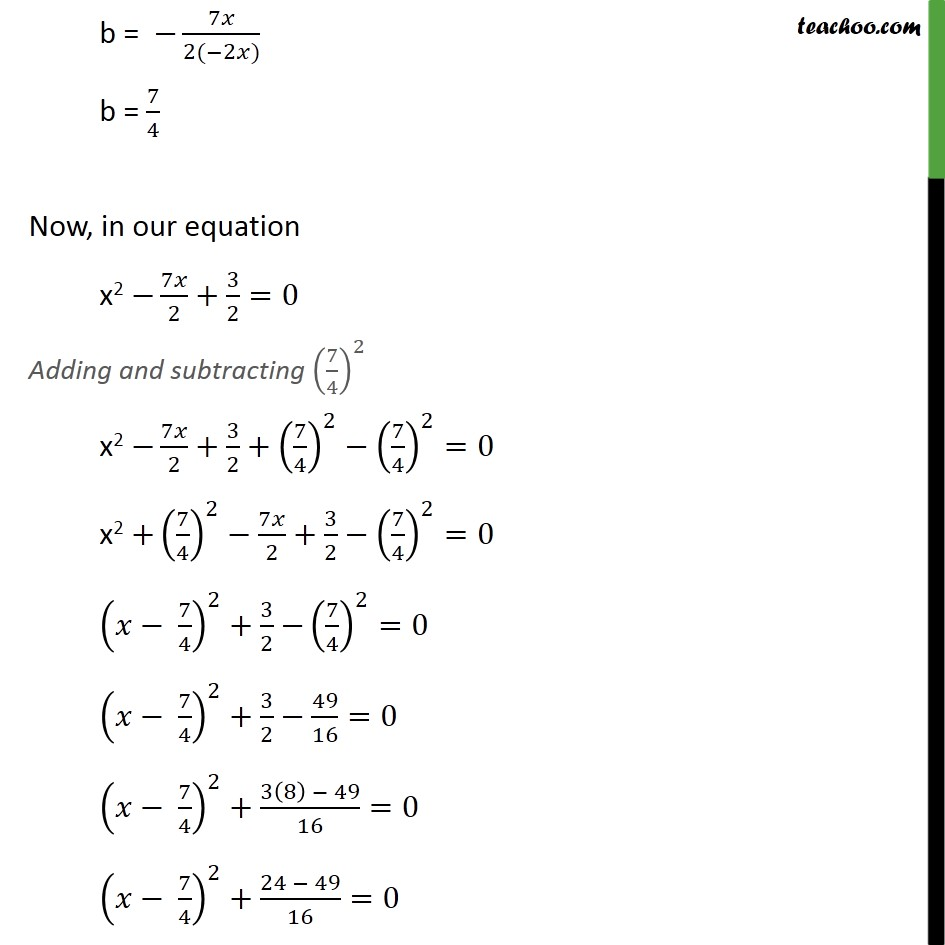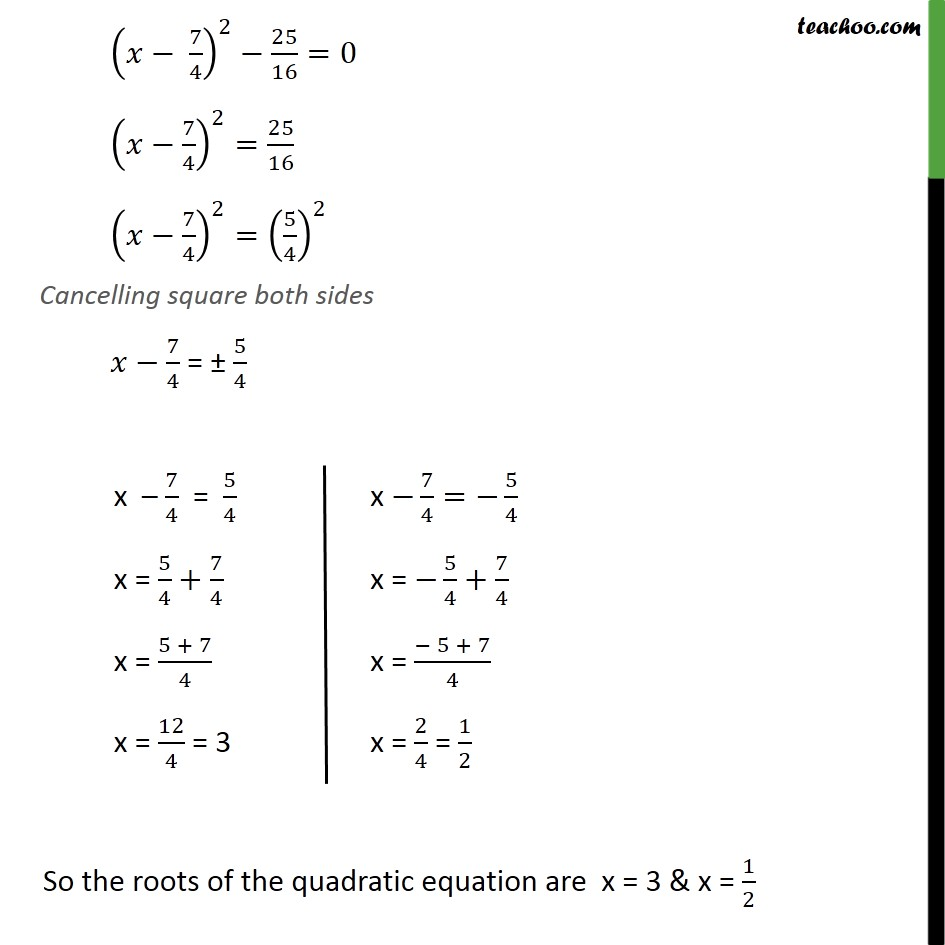1. Chapter 4 Class 10 Quadratic Equations (Term 2)
2. Concept wise
3. Solving by completing square

Transcript

Ex 4.3 ,1 Find the roots of the following quadratic equations, if they exist, by the method of completing the square: (i) 2x2 – 7x + 3 = 0 2x2 – 7x +3 = 0 Dividing by 2 (2𝑥2 − 7𝑥 + 3 = 0)/2=0/2 2𝑥2/2 – 7𝑥/2 + 3/2=0 x2 – 7𝑥/2+3/2=0 We know that (a – b)2 = a2 – 2ab + b2 Here, a = x & – 2ab = – 7𝑥/2 – 2xb = −7𝑥/2 b = −7𝑥/(2(−2𝑥)) b = 7/4 Now, in our equation x2 −7𝑥/2+3/2=0 Adding and subtracting (7/4)^2 x2 −7𝑥/2+3/2+(7/4)^2−(7/4)^2=0 x2 +(7/4)^2−7𝑥/2+3/2−(7/4)^2=0 (𝑥− 7/4)^2+3/2 −(7/4)^2=0 (𝑥− 7/4)^2+3/2−49/16=0 (𝑥− 7/4)^2+(3(8) − 49)/16=0 (𝑥− 7/4)^2+(24 − 49)/16=0 (𝑥− 7/4)^2−25/16=0 (𝑥−7/4)^2=25/16 (𝑥−7/4)^2=(5/4)^2 Cancelling square both sides 𝑥−7/4 = ± 5/4